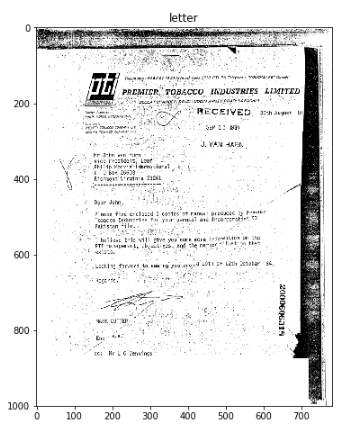# How I Built A Document Classification System using Deep Convolutional Neural Networks !

Source: Deep Learning on Medium## Creating Structured Data

The label files list the images and their categories in the following format:

`path/to/the/image.tif category`

We create a script such that our we get two separate pickel files for labels as well path to images

`import osimport joblibcount=0labels=[]directories=['C:/Users/sambal/Desktop/labels/train.txt','C:/Users/sambal/Desktop/labels/test.txt','C:/Users/sambal/Desktop/labels/val.txt']train_path=[]test_path=[]val_path=[]paths=[train_path,test_path,val_path]y_train=[]y_test=[]y_cv=[]labels=[y_train,y_test,y_cv]for i in range(3): label=[] with open(directories[i],'r') as f: for line in f: label.append(line)path=[]for x in label:category=x.split(" ")[-2::-1][::-1] labels[i].append(category) y=x.split(" ") paths[i].append(y)for i in range(3): print(paths[i],labels[i])joblib.dump(labels,"labels")joblib.dump(paths,"paths")`

Once we have out labels and paths separated into files we can now move forward and filter our data into three parts-Train,Test and Cv.

## Lets check if there is any variation in image sizes using some samples of image

`train_width=[]test_width=[]cv_width=[]count=0for image in train[:100]:im = cv2.imread(image,cv2.IMREAD_GRAYSCALE)train_width.append(im.shape)for image in test[:100]:im = cv2.imread(image,cv2.IMREAD_GRAYSCALE)test_width.append(im.shape)count+=1for image in cv[:100]:im = cv2.imread(image,cv2.IMREAD_GRAYSCALE)cv_width.append(im.shape)`

Lets visualize the image width distribution.

`import seaborn as sbfrom matplotlib import pyplot as pltwidth=(train_width,test_width,cv_width)for x in width: sb.distplot(x,kde = True) plt.show()`